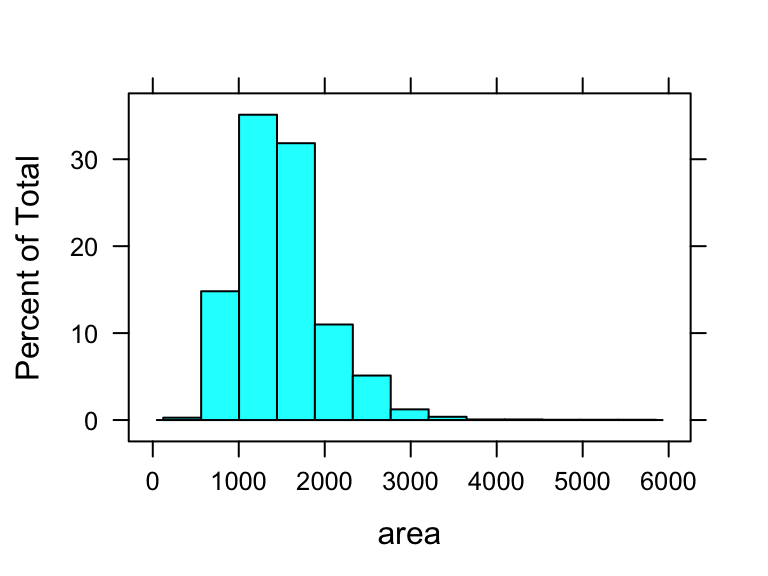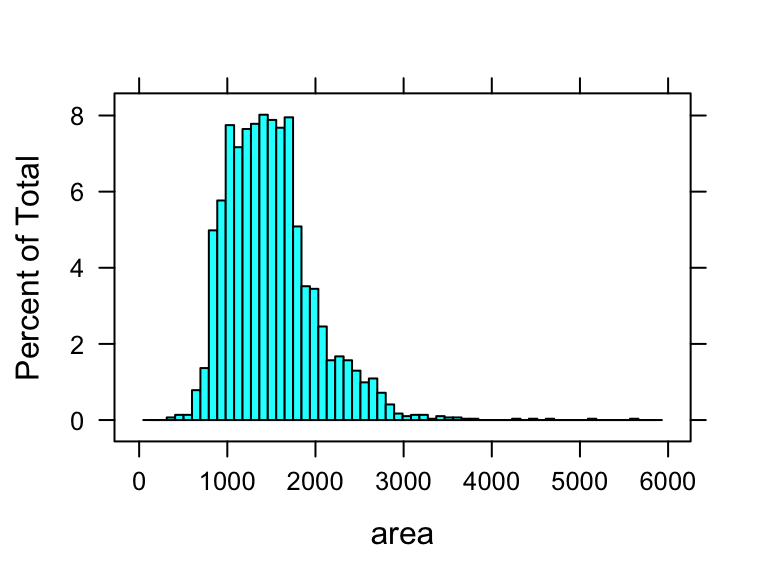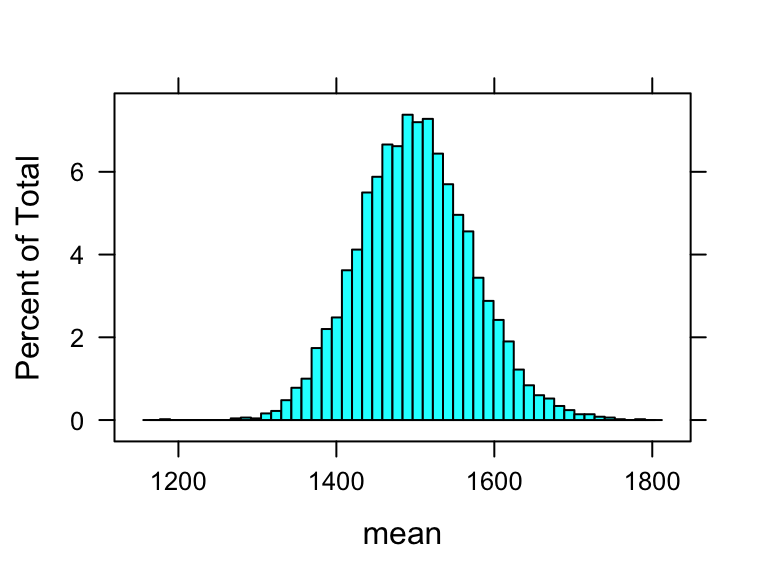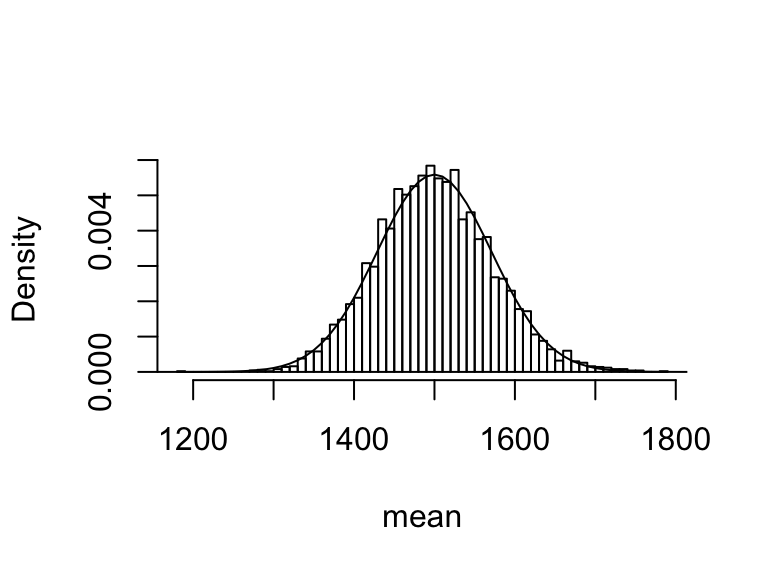In this lab, we will make sure of the lattice and tidyverse packages.

library(lattice)
library(tidyverse)

We will also make use of the following function that provides us with useful summaries:

favstats = function(mydata){
# input mydata is a numerical vector or matrices
result = rep(0, 9);
mysummary = summary(mydata);
result = mysummary;
result = mysummary;
result = mysummary;
result = mysummary;
result = mysummary;
result = mysummary;
result = sd(mydata);
result = length(mydata);
result = sum(is.na(mydata));
names(result) = c("min", "Q1", "median", "mean", "Q3", "max", "sd", "n", "missing");
result;
}

In this lab, we investigate the ways in which the statistics from a random sample of data can serve as point estimates for population parameters. We’re interested in formulating a sampling distribution of our estimate in order to learn about the properties of the estimate, such as its distribution.

## The Ames Real Estate Data

We consider real estate data from the city of Ames, Iowa. The details of every real estate transaction in Ames is recorded by the City Assessor’s office. Our particular focus for this lab will be all residential home sales in Ames between 2006 and 2010. This collection represents our population of interest. In this lab we would like to learn about these home sales by taking smaller samples from the full population. Let’s load the data.

download.file("http://www.openintro.org/stat/data/ames.RData", destfile = "ames.RData")
load("ames.RData")

We see that there are quite a few variables in the data set, enough to do a very in-depth analysis. (A description of all variables can be found at http://www.amstat.org/publications/jse/v19n3/decock/DataDocumentation.txt.) For this lab, we’ll focus our attention to just two of the variables: the above ground living area of the house in square feet (Gr.Liv.Area) and the sale price (SalePrice). To save some effort throughout the lab, create two variables with short names that represent these two variables.

area = ames$Gr.Liv.Area price = ames$SalePrice

Let’s look at the distribution of area in our population of home sales.

favstats(area)
##       min        Q1    median      mean        Q3       max        sd         n
##  334.0000 1126.0000 1442.0000 1499.6904 1742.7500 5642.0000  505.5089 2930.0000
##   missing
##    0.0000
histogram(area)If you would like to adjust the number of class intervals of your histogram, you can do so by specifying the nint (for number of intervals) argument.

histogram(area, nint=60)The command above gives a histogram with 60 class intervals. Try a number of different values of nint until you get a histogram that best depicts the shape of the distribution.

Exercise 1: Comment on the shape of this population distribution. Is it symmetric, left-skewed or right-skewed?

## The unknown sampling distribution

In this lab we have access to the entire population, but this is rarely the case in real life.
Gathering information on an entire population is often extremely costly or impossible.
Because of this, we often take a sample of the population and use that to understand the properties of the population.

If we were interested in estimating the mean living area in Ames based on a sample, can use the following command to survey the population.

samp1 = sample(area, 50)

This command collects a simple random sample of size 50 from the vector area, and then store the sample in the variable samp1.
This is like going into the City Assessor’s database and pulling up the files on 50 random home sales.
Working with these 50 files would be considerably simpler than working with all 2930 home sales.

Exercise 2: Describe the distribution of this sample. How does it compare to the distribution of the population?

If we’re interested in estimating the average living area in homes in Ames using the sample, our best single guess is the sample mean.

mean(samp1)

Depending on which 50 homes you selected, your estimate could be a bit above or a bit below the true population mean of 1499.69 square feet. In general, though, the sample mean turns out to be a pretty good estimate of the average living area, and we were able to get it by sampling less than 3% of the population.

Exercise 3: Take a second sample, also of size 50, and call it samp2. How does the mean of samp2 compare with the mean of samp1? Suppose we took two more samples, one of size 100 and one of size 1000. Which would you think would provide a more accurate estimate of the population mean?

Not surprisingly, every time we take another random sample, we get a different sample mean. It’s useful to get a sense of just how much variability we should expect when estimating the population mean this way. The distribution of sample means, called the sampling distribution, can help us understand this variability. In this lab, because we have access to the population, we can build up the sampling distribution for the sample mean by repeating the above steps many times.

Computing a single sample mean is easy:

samp = mean(sample(area, 50))

But how can we compute, say, 5000 sample means, and put those results into a data frame to get a sampling distribution? We can use the replicate function in R, which is a useful tool for repeating a procedure.

sample_means50 = as.data.frame(replicate(5000, mean(sample(area, 50))))
names(sample_means50) = "mean"
head(sample_means50)
##      mean
## 1 1345.84
## 2 1445.50
## 3 1561.68
## 4 1495.90
## 5 1484.46
## 6 1580.90

We can examine the sampling distribution from the summary statistics and the histogram.

favstats(sample_means50$mean) ## min Q1 median mean Q3 max sd ## 1187.56000 1451.52500 1498.33000 1499.87481 1546.10500 1780.10000 71.38532 ## n missing ## 5000.00000 0.00000 histogram(sample_means50$mean, nint=50, xlab="mean")Again you should try a number of different nint values until you get a histogram that best displays the shape of the distribution. You can overlay a normal density on the histogram using the same approach in Lab 5.

hist(sample_means50$mean, breaks = 50, freq=F, xlab="mean", main='') x = seq(1000, 2000, by=10); y = dnorm(x, mean(sample_means50$mean),
sd(sample_means50$mean)) lines(x, y)Exercise 4: How many elements are there in sample_means50? Describe the sampling distribution, and be sure to specifically note its center. Would you expect the distribution to change if we instead collected 50,000 sample means? ## Interlude: The replicate function Let’s take a break from the statistics for a moment to let that last block of code sink in. The idea behind the replicate function is repetition: it allows you to execute a line of code as many times as you want and put the results in a data frame. In the case above, we wanted to repeatedly take a random sample of size 50 from area and then save the mean of that sample into the sample_means50 vector. Without the replicate function, this would be painful. First, we’d have to create an empty vector filled with 0s to hold the 5000 sample means. Then, we’d have to compute each of the 5000 sample means one line at a time, putting them individually into the slots of the sample_means50 vector: sample_means50 = rep(NA, 5000) sample_means50 = mean(sample(area, 50)) sample_means50 = mean(sample(area, 50)) sample_means50 = mean(sample(area, 50)) sample_means50 = mean(sample(area, 50)) # ...and so on, 5000 times With the replicate function, these thousands of lines of code are compressed into one line: sample_means10 = as.data.frame(replicate(5000, mean(sample(area, 10)))) Note that for each of the 5000 times we computed a mean, we did so from a different sample! Exercise 5: To make sure you understand what the replicate function does, try modifying the code to take only 100 sample means and put them in a data frame named sample_means_small. Print the output to your screen (type sample_means_small into the console and press enter). How many elements are there in this object called sample_means_small? What does each element represent? ## Sample size and the sampling distribution Mechanics aside, let’s return to the reason we used the replicate function: to compute a sampling distribution. The sampling distribution that we computed tells us much about estimating the average living area in homes in Ames. Because the sample mean is an unbiased estimator, the sampling distribution is centered at the true average living area of the the population, and the spread of the distribution indicates how much variability is induced by sampling only 50 home sales. To get a sense of the effect that sample size has on our distribution, let’s build up three sampling distributions: one based on a sample size of 10, one based on a sample size of 50, and another based on a sample size of 100. sample_means10 = as.data.frame(replicate(5000, mean(sample(area, 10)))) names(sample_means10) = "mean" sample_means50 = as.data.frame(replicate(5000, mean(sample(area, 50)))) names(sample_means50) = "mean" sample_means100 = as.data.frame(replicate(5000, mean(sample(area, 100)))) names(sample_means100) = "mean" hist(sample_means10$mean, breaks = 50, freq=F, xlab="mean", main="")
x = seq(1000, 2000, by=10);
y = dnorm(x, mean(sample_means10$mean), sd(sample_means10$mean))
lines(x, y)

hist(sample_means50$mean, breaks = 50, freq=F, xlab="mean", main="") x = seq(1000, 2000, by=10); y = dnorm(x, mean(sample_means50$mean), sd(sample_means50$mean)) lines(x, y) hist(sample_means100$mean, breaks = 50, freq=F, xlab="mean", main="")
x = seq(1000, 2000, by=10);
y = dnorm(x, mean(sample_means100$mean), sd(sample_means100$mean))
lines(x, y)
1. Make a histogram for each to examine the three sampling distributions. When the sample size is larger, what happens to the center, spread, and shape of the sampling distributions?

## Using the Central Limit Theorem

From the histogram above, we can see when the sample size is 100, the sampling distribution of the sample mean is fairly close to normal. Based on the Central Limit Theorem, the sampling distribution of the sample mean is roughly $$N(\mu,\sigma/sqrt{n})$$, where $$\mu=1499.69$$ Sq.ft. is the population mean and $$\sigma=505.5089$$ Sq.ft. is the population SD. The values of $$\mu$$ and $$\sigma$$ have been found in the beginning.

As the sampling distribution of the sample mean looks fairly normal when the sample size is 100, we can use the CLT to find the (approximate) probability of getting a sample mean below 1450 Sq. ft. That is, to find $$P(\overline{X}<1450)$$ where $$\overline{X}$$ is normal with mean $$\mu=1499.69$$ and SD $$\sigma/\sqrt{n}=505.5089/\sqrt{100}=50.55089.$$ The z-score for 1450 is $$Z=(1450-1499.69)/50.55089\approx-0.983.$$ The probability is hence $P(\overline{X}<1450)\approx P(Z<-0.98)\approx 0.1635\approx 16\%$ So drawing a random sample of size 100, the sample mean will be below 1450 for about 16% of the time.

Alternatively, we can compute the probability above using R as follows

pnorm(1450, 1499.69, 505.5089/sqrt(100))

We can check how CLT works by checking how close the probability computed above is to the actual proportion of the 5000 sample means that are below 1450. The R commands below count the number of entries in sample_means100 that are $$<1450$$ (TRUE) and $$\ge 1450$$ (FALSE), and then convert the counts to proportions.

table(sample_means100 < 1450)
table(sample_means100 < 1450)/5000

Is the proportion close to 16%?

So far, we have only focused on estimating the mean living area in homes in Ames. Now you’ll try to estimate the mean home price.

• Make a histogram of price, which shows the population distribution of the sale price of all 2930 homes in the data. Comment on the shape of the histogram.
• Find the mean and SD of price, which are the population mean and the population SD.
• Take a random sample of size 25 from price. Find the sample mean, and compared it with the population mean you found the previous part
• Since you have access to the population, simulate the sampling distribution for $$\bar{x}_{price}$$ by taking 5000 samples from the population of size 25 and computing 5000 sample means. Store these means in a vector called sample_means25. Make a histogram of the 5000 sample means.
• Repeat the previous part but change the sample size from 25 to 4. Store the 5000 sample means in a vector called sample_means4.
• Repeat the previous part again but change the sample size to 100. Store the 5000 sample means in a vector called sample_means100.
• Compare the center, spread, and the shape Of the 3 sampling distributions from previous 3 parts. How do the center, spread, and shape of the sampling distributions change with the sample size?
• Theoretically, the mean and the SD of the sampling distribution are respectively $$\mu$$ and $$\sigma/\sqrt{n}$$, where $$\mu$$ and $$\sigma$$ are the population mean and the population SD found in part (b), and $$n$$ is the sample size.

Compute the means and SDs for the 5000 sample means as follows.

mean(sample_means4$mean) mean(sample_means25$mean)
mean(sample_means100$mean) sd(sample_means4$mean)
sd(sample_means25$mean) sd(sample_means100$mean)

Are they close to their respective theoretical values $$\mu$$ and $$\sigma/\sqrt{n}$$?

• Use the CLT to find the (approximate) probability of getting a sample mean below $170,000, when the sample size is 100. • Find the percentage of the 5000 sample means in sample_means100$mean that are below $170,000. Is the percentage close to the probability computed in the previous part using CLT? • Use the CLT to find the (approximate) probability of getting a sample mean between$130,000, and $190,000, when the sample size is 4. • Find the percentage of the 5000 sample means in sample_means4$mean that are between $130,000, and$190,000.
table(sample_means100 < 190000 & sample_means100 > 130000)
table(sample_means100 < 190000 & sample_means100 > 130000)/5000

Is the percentage close to the probability computed in the previous part using CLT? Does the CLT work well when the sample size is only 4?

### Disclaimer

This lab was expanded for STAT 220 by Yibi Huang from a lab released from OpenIntro by Andrew Bray and Mine Çetinkaya-Rundel, which originally was based on a lab written by Mark Hansen of UCLA Statistics.

This lab can be shared or edited under a Creative Commons Attribution-ShareAlike 3.0 Unported licence.